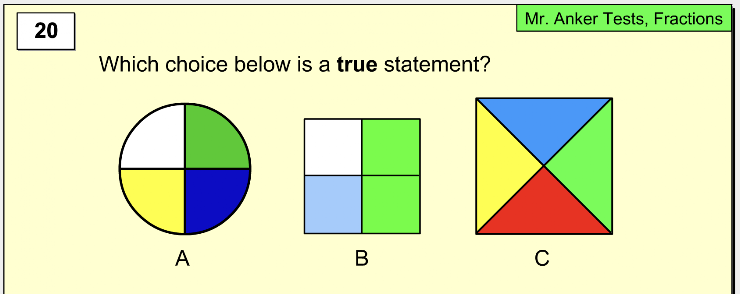Fractions 4
Set 4 (20 Questions)
Students understand that fractions and decimals may refer to parts of a set and parts of a whole.
Recognize, name, and compare unit fractions from 1/12 to 1/2.
Recognize fractions of a whole and parts of a group (e.g., one-fourth of a pie, two thirds of 15 balls).
From Mr. Anker Tests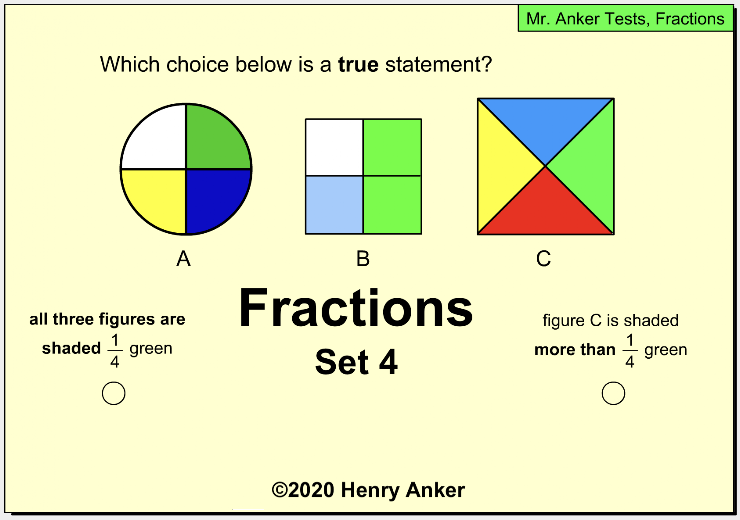1. Which fraction below is equal in value to the shaded area of the circle? *
1 point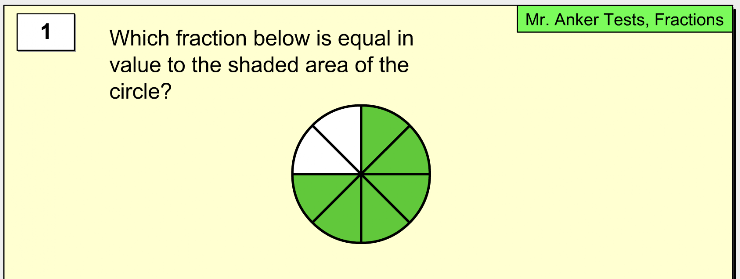2. Which picture has a shaded region greater than 1/2? *
1 point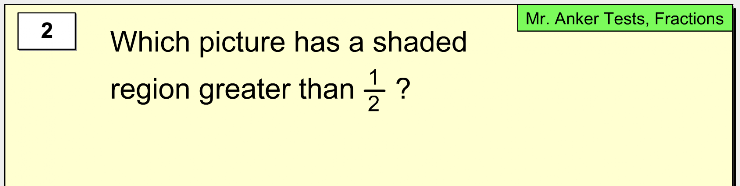3. What fraction of the whole figure is shaded? *
1 point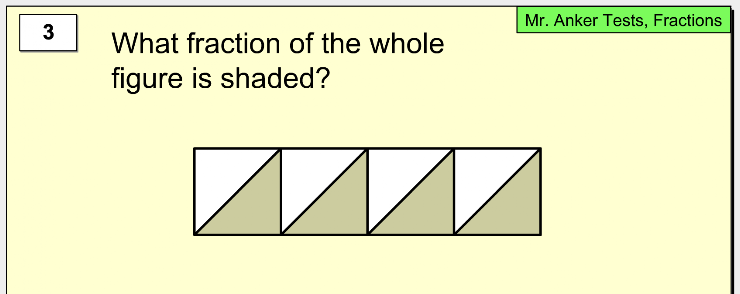4. What fraction of the shapes below are white? *
1 point5. What fraction of the shapes below are triangles? *
1 point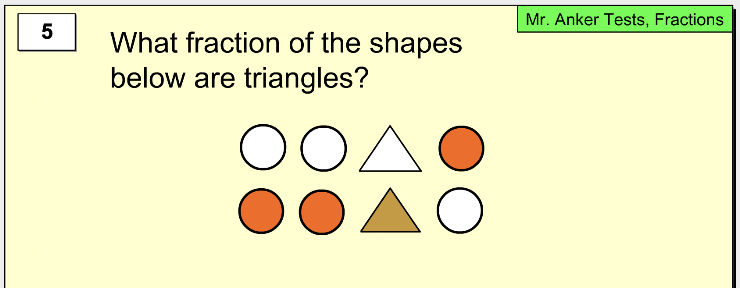6. What fraction of this circle is not shaded? *
1 point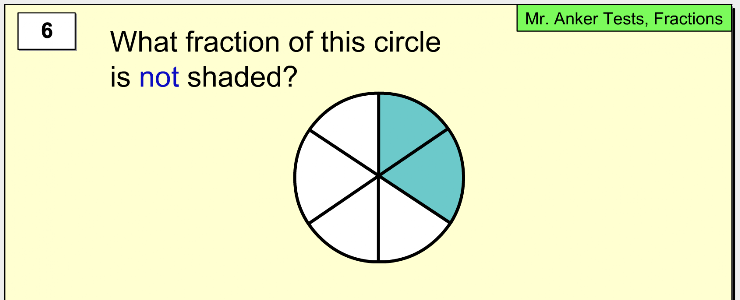7. What fraction of this rectangle is shaded? *
1 point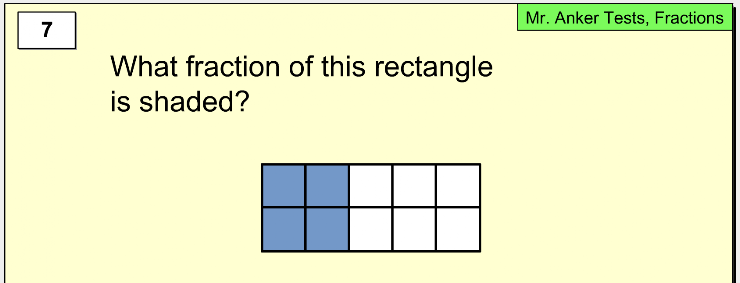8. Which fraction of a dollar is shown? *
1 point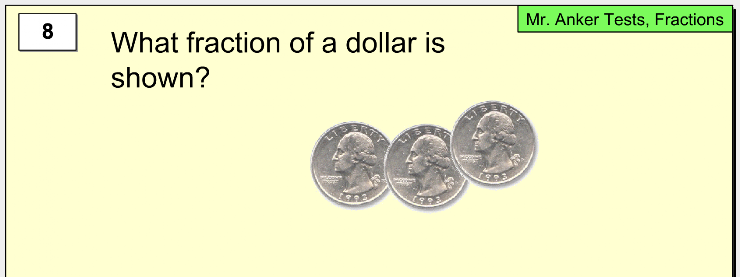9. Which fraction of this rectangle is shaded purple? *
1 point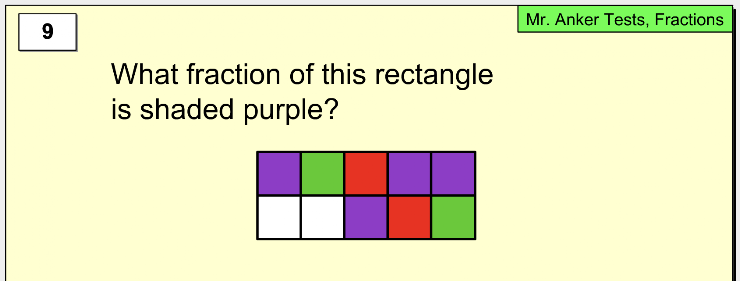10. What fraction of the square is not green or blue? *
1 point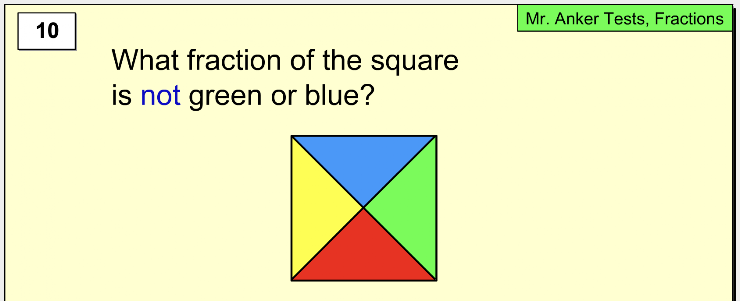11. What fraction of the circles are shaded orange? *
1 point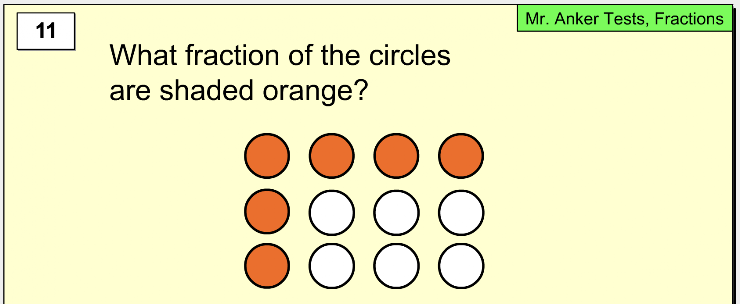12. What fraction of the circles are shaded yellow? *
1 point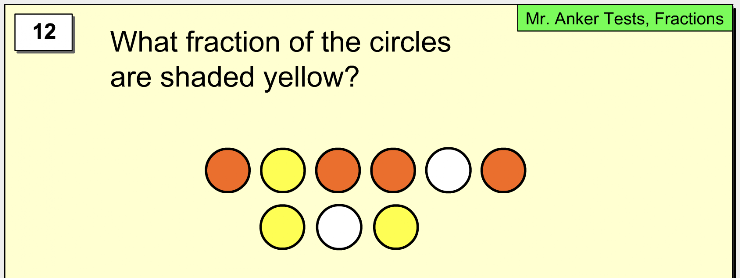13. What fraction of the arrows are pointing up? *
1 point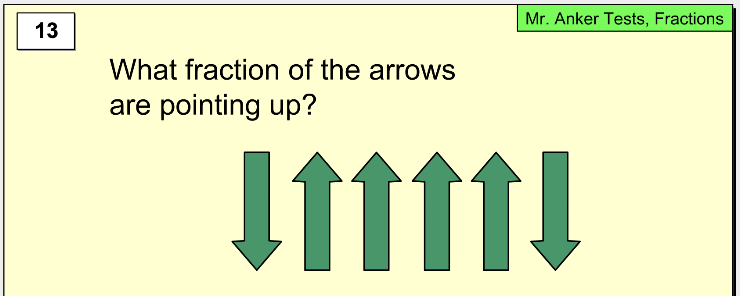14. What fraction of the faces are happy? *
1 point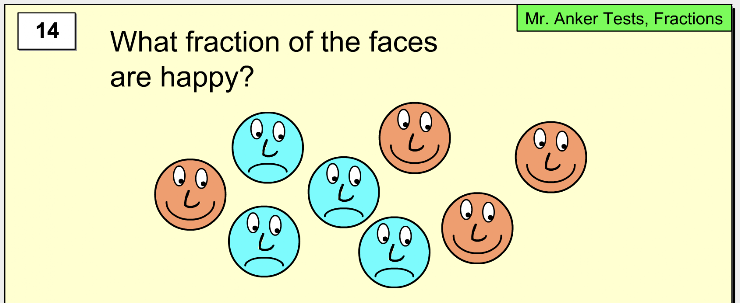15. Which fraction reflects approximately how much of the glass is filled? *
1 point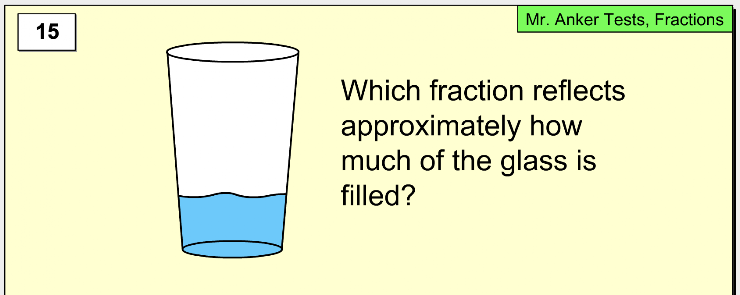16. What fraction of the pencils are in need of sharpening? *
1 point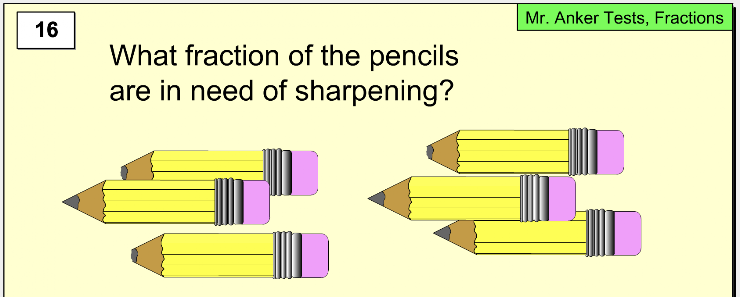17. Twenty-five cents is what fraction of one dollar? *
1 point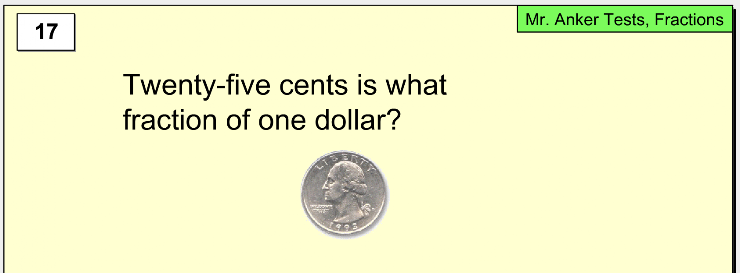18. Which rectangle has 1/3 of its area shaded in? *
1 point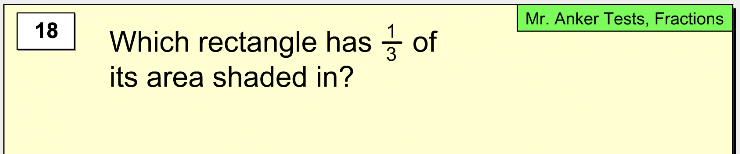19. Which rectangle has 3/4 of its area shaded in? *
1 point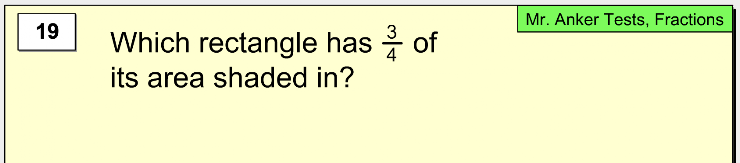20. Which choice below is a true statement? *
1 point